# Rabbit family

Rabbit family ate 32 pieces of carrots, small one ate 6 pieces, dad 5 more than the mother. How much ate mom?

x =  10.5

### Step-by-step explanation:

32 = 6 + x + (x+5)

2x = 21

x = 21/2 = 10.5

Our simple equation calculator calculates it.Did you find an error or inaccuracy? Feel free to write us. Thank you!Tips to related online calculators
Do you have a linear equation or system of equations and looking for its solution? Or do you have a quadratic equation?

## Related math problems and questions:

• Family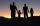Martin has just as brothers as sisters. His sister Jana but has 2 times more brothers than sisters. a) How many children are in this family? b) How many boys and how many girls are in the family?
• Two pizzasJacobs mom bought two whole pizzas. He ate 2/10 of the pizza and his dad ate 1 1/5. How much is left.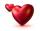Mom wants talk with dad 2.2 hours. But dad wants of 2 times more hours than mom ... How many hours wants dad talk with mom? ...
• Birds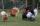On the farm they have a total of 110 birds. Geese and turkeys together is 47. Hens is three times more than the turkey. How much is poultry by species?
• FamilySon is 3/5 smaller than the mother. Mother is 99 cm higher than the son. How tall is the mother?
• Classroom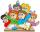In a class are 32 pupils. Boys are 8 less than girls. How many boys and girls are in the classroom?
• Family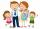Dad has two years more than mom. Mom has 5 times more than Katy. Katy has 2 times less than Jan. Jan is 10 years old. How old is everyone in the family? How old are all together?
• Roma ateRoma ate 2/5 of a cake while Somya ate 3/7 of the same cake. Who ate more and by how much?
• Czech crowns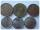Small Petra save money. Grandfather give her 10 crowns. Petra asked his older sister let her calculate how much he has saved up. Sister told her that he has less than 30 crowns. If the sum is divided by three left one crown. If the sum is divided by the f
• Dividing moneyVilem, Cenek, and Edita divided the money they earned by spreading the leaflet. Vilem got 240 CZK more than Cenek and twice more than Edita. Edita got 400kc less than Vilem.
• Buns and toastsTwo buns weigh 10 grams more than two toasts. One Bun and two Toast weigh a total of 110 grams. How many grams weigh three toasts? How many grams does a Bun weigh?
• One frame5 picture frames cost € 12 more than three frames. How much cost one frame?
• Family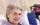Mom is 39 years old. Karl is eight years and Paul 4 years. For many years mother would old just like two children together?
• HomeworkMother and Kate together weigh 24.5 kg more than the father. Father weighs 7.6 kg more than mother. Father weight 44.8 kg more than Kate. Determine weights of father mother and Kate.
• The average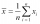The average of one set of 4 numbers is 35. The average of another set of number is 20. The average of the numbers in the two sets is 30. How many numbers are there in the other set?
• Dinosaurs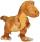More than 30 and less than 60 dinosaurs have met at the pond. A quarter of them bathed and 1/7 saws, and the rest gripped. How many were at the pond? How many were there?
• Bob and Bobek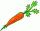Bobek has 2 cabbages and 5 carrots, Bob has 2 cabbages and 9 carrots. When Bobek stepped on the scale weight 5800 g and when Bob weight 8960 g. Actual Bob weight is 8 kg and Bobek 5 kg. How much weighs one carrot?